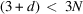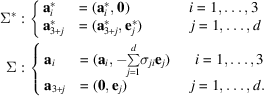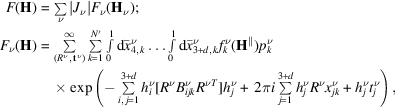International
Tables for
Crystallography
Volume B
Reciprocal space
Edited by U. Shmueli

International Tables for Crystallography (2010). Vol. B, ch. 4.6, pp. 602-603   | 1 | 2 |

## Section 4.6.3.2. Composite structures (CSs)

W. Steurera* and T. Haibacha

aLaboratory of Crystallography, Department of Materials, ETH Hönggerberg, HCI G 511, Wolfgang-Pauli-Strasse 10, CH-8093 Zurich, Switzerland
Correspondence e-mail:  walter.steurer@mat.ethz.ch

#### 4.6.3.2. Composite structures (CSs)

| top | pdf |

Composite structures consist of N mutually incommensurate substructures with N basic sublattices, with. The reciprocal sublattices, with, have either only the origin of the reciprocal lattice or one or two reciprocal-lattice directions in common. Thus, one needsreciprocal-basis vectors for integer indexing of diffraction patterns that show Bragg reflections at positions given by the Fourier module. The CSs discovered to date have at least one lattice direction in common and consist of a maximum number ofsubstructures. They can be divided in three main classes: channel structures, columnar packings and layer packings (see van Smaalen, 1992, 1995).

In the following, the approach of Janner & Janssen (1980b)and van Smaalen (1992, 1995, and references therein) for the description of CSs is used. The set of diffraction vectors of a CS, i.e. its Fourier module, can be split into the contributions of the ν subsystems by employingmatriceswith integer coefficients,. In the general case, each subsystem will be modulated with the periods of the others due to their mutual inter­actions. Thus, in general, CSs consist of several intergrown incommensurately modulated substructures. The satellite vectors, referred to the νth subsystem can be obtained fromby applying theinteger matrices:,. The matrices consisting of the componentsof the satellite vectorswith regard to the reciprocal sublatticescan be calculated by, where the subscript 3 refers to thesubmatrix of physical space and the subscript d to thematrix of the internal space. The juxtaposition of thematrixand thematrixdefines the non-singularmatrix,This matrix allows the reinterpretation of the Fourier moduleas the Fourier moduleof a d-dimensionally modulated subsystem ν. It also describes the coordinate transformation between the superspace basis Σ and.

The superspace description is obtained analogously to that for IMSs (see Section 4.6.3.1) by considering the 3D Fourier moduleof rankas the projection of areciprocal latticeupon the physical space. Thus, one obtains for the definition of the direct and reciprocallattices (Janner & Janssen, 1980b)#### 4.6.3.2.1. Indexing

| top | pdf |

The indexing of diffraction patterns of composite structures can be performed in the following way:

 (1) find the minimum number of reciprocal latticesnecessary to index the diffraction pattern; (2) find a basis for, the union of sublattices; (3) find the appropriate superspace embedding.

Thevectorsforming a basis for the 3D Fourier modulecan be chosen such that,andare linearly independent. Then the remaining d vectors can be described as a linear combination of the first three, defining thematrix σ:. This is formally equivalent to the reciprocal basis obtained for an IMS (see Section 4.6.3.1) and one can proceed in an analogous way to that for IMSs.

#### 4.6.3.2.2. Diffraction symmetry

| top | pdf |

The symmetry of CSs can be described with basically the same formalism as used for IMSs. This is a consequence of the formally equivalent applicability of the higher-dimensional approach, in particular of the superspace-group theory developed for IMSs [see Janner & Janssen (1980a,b); van Smaalen (1991, 1992); Yamamoto (1992a)].

#### 4.6.3.2.3. Structure factor

| top | pdf |

The structure factorof a composite structure consists of the weighted contributions of the subsystem structure factors:with coefficients similar to those for IMSs.

The weights are the Jacobians of the transformations fromto t, andare the reflection indices with respect to the subsystem Fourier modules(van Smaalen, 1995, and references therein). The relative values of, whereare related to the volume ratios of the contributing subsystems. The subsystem structure factors correspond to those for IMSs (see Section 4.6.3.1). Besides this formula, based on the publications of Yamamoto (1982)and van Smaalen (1995), different structure-factor equations have been discussed (Kato, 1990; Petricek, Maly, Coppens et al., 1991).

### References

Janner, A. & Janssen, T. (1980a). Symmetry of incommensurate crystal phases. I. Commensurate basic structures. Acta Cryst. A36, 399–408.
Janner, A. & Janssen, T. (1980b). Symmetry of incommensurate crystal phases. II. Incommensurate basic structures. Acta Cryst. A36, 408–415.
Kato, K. (1990). Strukturverfeinerung des Kompositkristalls im mehr­dimensionalen Raum. Acta Cryst. B46, 39–44.
Petricek, V., Maly, K., Coppens, P., Bu, X., Cisarova, I. & Frost-Jensen, A. (1991). The description and analysis of composite crystals. Acta Cryst. A47, 210–216.
Smaalen, S. van (1991). Symmetry of composite crystals. Phys. Rev. B, 43, 11330–11341.
Smaalen, S. van (1992). Superspace description of incommensurate intergrowth compounds and the application to inorganic misfit layer compounds. Mater. Sci. Forum, 100 & 101, 173–222.
Smaalen, S. van (1995). Incommensurate crystal structures. Crystallogr. Rev. 4, 79–202.
Yamamoto, A. (1982). Structure factor of modulated crystal structures. Acta Cryst. A38, 87–92.
Yamamoto, A. (1992a). Unified setting and symbols of superspace groups for composite crystals. Acta Cryst. A48, 476–483.